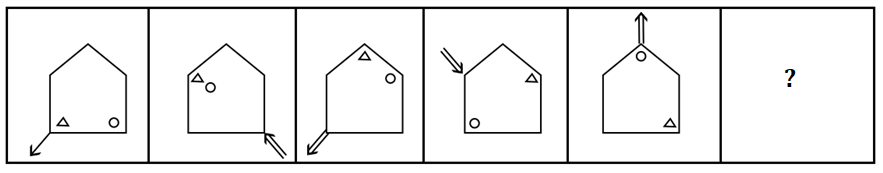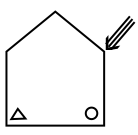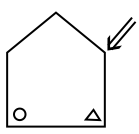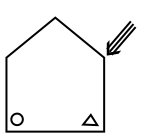# Which one of the following will be the missing figure in the below arrangement which will maintain the essence of the arrangement?

2019-09-12 | Team PendulumEduOptions:

A.B.C.D.Solution:

The logic of the above series can be defined as

Logic 1: Triangle in the figure is moved by one point in the clockwise direction, and Circle is moved two points in the clockwise direction to obtain the next figure in the series.

Logic 2: If circle and Triangle come in the same place, then the number of lines in the arrow is increased by one.

Logic 3: To obtain the next figure in the figure series, the arrow is moved to the next position in the clockwise direction and direction of the arrow is changed in the opposite direction. When the circle and triangle are in the same position, then the arrow is shifted to the fourth-place clockwise.

From the above logic, we can say that the next figure in the figure series will beHence, (C) is the correct answer.

Such type of question is asked in the Logical Reasoning section of various exams like SSC CGL, SSC MTS, SSC CPO, SSC CHSL, RRB JE, RRB NTPC, RRB GROUP D, etc. Try and attempt free mock tests at PendulumEdu and learn easy methods to solve logical reasoning questions in less time.0